# Maharashtra Board Class 8 Maths Solutions Chapter 14 Compound Interest Practice Set 14.2

## Maharashtra State Board Class 8 Maths Solutions Chapter 14 Compound Interest Practice Set 14.2

Compound Interest class 8 practice set 14.2 Question 1. On the construction work of a flyover bridge there were 320 workers initially. The number of workers were increased by 25% every year. Find the number of workers after 2 years.
Solution:
Here, P = Initial number of workers = 320
R = Increase in the number of workers per year = 25%
N = 2 years
A = Number of workers after 2 years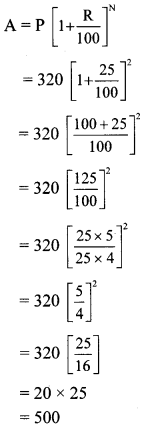∴ The number of workers after 2 years would be 500.

Question 2.
A shepherd has 200 sheep with him. Find the number of sheeps with him after 3 years if the increase in number of sheeps is 8% every year.
Solution:
Here, P = Present number of sheeps = 200
R = Increase in number of sheeps per year = 8%
N = 3 years
A = Number of sheeps after 3 years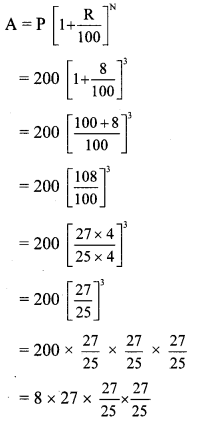= $$\frac{0.32}{25} \times 27 \times 27 \times 27$$
= 0.0128 × 27 × 27 × 27
= 251.9424
= 252
∴ The number of sheeps with the shepherd after 2 years would be 252 (approx).

8th Class Math Practice Set 14.2 Question 3.
In a forest there are 40,000 trees. Find the expected number of trees after 3 years if the objective is to increase the number at the rate 5% per year.
Solution:
Here, P = Present number of trees in the forest = 40,000
R = Increase in the number of trees per year = 5%
N = 3 years
A = Number of trees after 3 years= 5 × 21 × 21 × 21
= 5 × 9261
= 46,305
∴ The expected number of trees in the forest after 3 years is 46,305.

Std 8 Maths Practice Set 14.2 Question 4.
The cost price of a machine is Rs 2,50,000. If the rate of depreciation is 10% per year, find the depreciation in price of the machine after two years.
Solution:
Here, P = Cost price of machine = Rs 2,50,000
R = Rate of depreciation per year = 10%
N = 2 years
A = Depreciated price of the machine after 2 years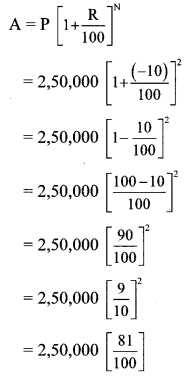= 2,500 × 81
= Rs 2,02,500
Depreciation in price = Cost price (P) – Depreciated price (A)
= 2,50,000 – 2,02,500
= Rs 47,500
∴ The depreciation in price of the machine after 2 years would be Rs 47,500.

Question 5.
Find the compound interest if the amount of a certain principal after two years is Rs 4036.80 at the rate of 16 p.c.p.a.
Solution:
Here, A = Rs 4036.80, R = 16 p.c.p.a. and N = 2 years
i. $$\mathbf{A}=\mathbf{P}\left[1+\frac{\mathbf{R}}{100}\right]^{N}$$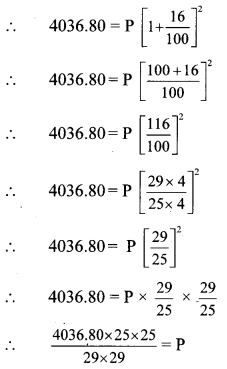ii. Interest = Amount (A) – Principal (P)
= 4036.80 – 3000
= Rs 1036.80
∴ The compound interest after 2 years would be Rs 1036.80.

Question 6.
A loan of Rs 15,000 was taken on compound interest. If the rate of compound interest is 12 p.c.p.a. find the amount to settle the loan after 3 years.
Solution:
Here, P = Rs 15,000, R = 12 p.c.p.a, and
N = 3 years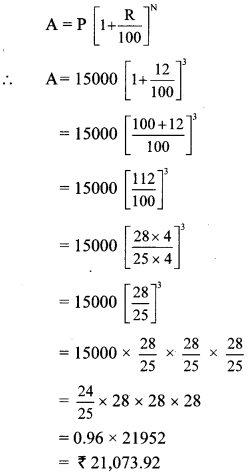∴ The amount required to settle the loan after 3 years is Rs 21,073.92.

Practice Set 14.2 Class 8 Question 7.
A principal amounts to Rs 13,924 in 2 years by compound interest at 18 p.c.p.a. Find the principal.
Solution:
Here, A = Rs 13,924, R = 18 p.c.p.a., and N = 2 years∴ P = 4 x 50 x 50
∴ P = Rs 10,000
∴ The principal is Rs 10,000.

Question 8.
The population of a suburb is 16,000. Find the rate of increase in the population if the population after two years is 17,640.
Solution:
Here, P = Population of a suburb = 16,000
N = 2 years
A = Increase in the population after 2 years = 17,640
R = Rate of increase in population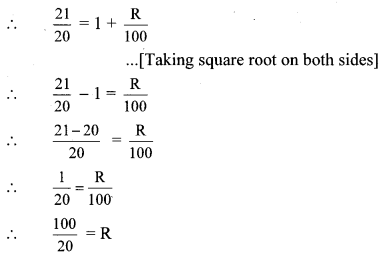∴5 = R
i.e., R = 5%
∴The rate of increase in the population is 5 p.c.p.a.

Compound Interest Practice Set 14.2 Question 9.
In how many years Rs 700 will amount to Rs 847 at a compound interest rate of 10 p.c.p.a.
Solution:
Here, P = Rs 700, R = 10 p.c.p.a., A = Rs 847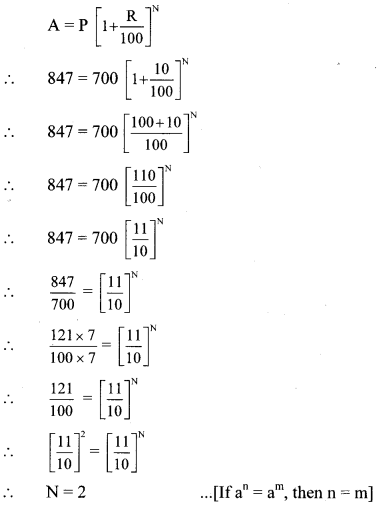∴Rs 700 will amount to Rs 847 in 2 years.

Practice Set 14.2 Question 10.
Find the difference between simple interest and compound interest on Rs 20,000 in 2 years at 8 p.c.p.a.
Solution:
Here, P = Rs 20,000, R = 8 p.c.p.a.,
N = 2 years
i. Simple interest (I)Simple interest (I) = Rs 3200

ii. Compound Interest (I):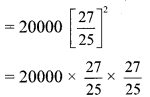= 32 × 27 × 27
= Rs 23,328
Compound interest (I)
= Amount (A) – Principal (P)
= 23,328 – 20,000
= Rs 3328 ,..(ii)

iii. Difference
= Compound interest – Simple interest
= 3328 – 3200 … [Form (i) and (ii)]
= Rs 128
∴ The difference between compound interest and simple interest is Rs 128.
[Note: The question is modified as per the answer given in the textbook.]

Maharashtra Board Class 8 Maths Chapter 14 Compound Interest Practice Set 14.2 Intext Questions and Activities

8th Standard Maths Practice Set 14.2 Question 1.
Visit the bank nearer to your house and get the information regarding the different schemes and rates of interests. Make a chart and display in your class. (Textbook pg. no. 90)
Solution:
(Students should attempt this activity at their own.)